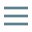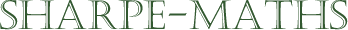# Glossary of terms

## F

•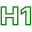### For all ($\forall$)

•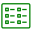For all values of a variable.

•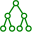The function $f$ is defined as $f(x)=\sqrt{x}, \ \forall x \in \mathbb{R}^+$

•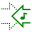•### Fraction

•A fraction is a mathematical symbol form which shows rational numbers in the form of an integer divided by another integer. It is okay to consider a fraction as being another way of showing a division operation.

•$2 \div 3 \equiv \frac{2}{3}$
•